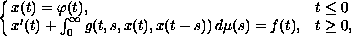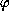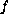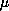Electron. J. Diff. Eqns., Vol. 1997(1997), No. 24, pp. 1-20.

### Initial value problems for nonlinear nonresonant delay differential equations with possibly infinite delay Lance D. Drager & William Layton

Abstract:
We study initial value problems for scalar, nonlinear, delay differential equations with distributed, possibly infinite, delays. We consider the initial value problemwhereandare bounded andis a finite Borel measure. Motivated by the nonresonance condition for the linear case and previous work of the authors, we introduce conditions on. Under these conditions, we prove an existence and uniqueness theorem. We show that under the same conditions, the solutions are globally asymptotically stable and, ifsatisfies an exponential decay condition, globally exponentially asymptotically stable.

Submitted August 14, 1997. Published December 19, 1997.
Math Subject Classification: 34K05, 34K20, 34K25
Key Words: Delay differential equation, infinite delay, initial value problem, nonresonance, asymptotic stability, exponential asymptotic stability.

Show me the PDF file (204 KB), TEX file, and other files for this article.

Lance D. Drager
Department of Mathematics and Statistics; Texas Tech University; Lubbock, TX 79409-1042 USA.
e-mail: drager@math.ttu.edu

William Layton
Department of Mathematics; University of Pittsburgh; Pittsburgh, PA 15260 USA.
e-mail: wjl+@pitt.edu